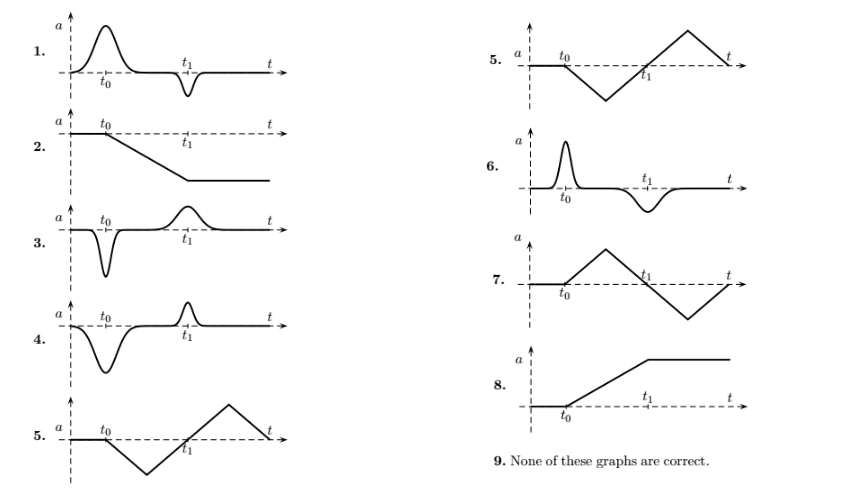Physics Practice Problems Velocity & Acceleration Graphs Practice Problems Solution: Henry hits a hockey puck in the positive x-directi...

🤓 Based on our data, we think this question is relevant for Professor Marder's class at TEXAS.

# Solution: Henry hits a hockey puck in the positive x-direction at time, t ≈ t  0. The puck is then stopped by a net starting at time, t ≈ t1. Which of the following curves could describe the acceleration of the hockey puck if we ignore any effects of friction?

###### Problem

Henry hits a hockey puck in the positive x-direction at time, t ≈ t  0. The puck is then stopped by a net starting at time, t ≈ t1. Which of the following curves could describe the acceleration of the hockey puck if we ignore any effects of friction?Velocity & Acceleration Graphs

Velocity & Acceleration Graphs

#### Q. A jogger starts from the origin and jogs in a straight line with the velocity profile shown below. [a] What is the maximum acceleration of the jogger...

Solved • Wed May 31 2017 06:02:42 GMT-0400 (EDT)

Velocity & Acceleration Graphs

#### Q. A block with an initial velocity v 0 slides up and back down a frictionless incline. Which graph best represents a description of the velocity of the ...

Solved • Wed May 17 2017 12:47:23 GMT-0400 (EDT)

Velocity & Acceleration Graphs

#### Q. Figure 2.2 shows the velocity time graph of a motorist during a 8 second period. The motorist leaves home (position x = 0m) at time t = 0s and the vel...

Solved • Wed Jan 18 2017 14:34:58 GMT-0500 (EST)

Velocity & Acceleration Graphs

#### Q. Figure 2.2 shows the velocity time graph of a motorist during a 8 second period. The motorist leaves home (position x = 0m) at time t = 0s and the vel...

Solved • Wed Jan 18 2017 14:23:30 GMT-0500 (EST)